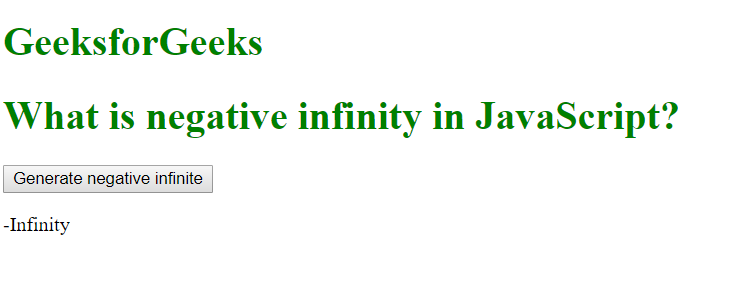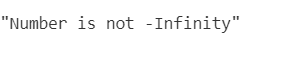Related Articles

# What is negative infinity in JavaScript?

• Last Updated : 30 Aug, 2019

The negative infinity in JavaScript is a constant value which is used to represent a value which is the lowest available. This means that no other number is lesser than this value. It can be generated using a self-made function or by an arithmetic operation.

Note: JavaScript shows the NEGATIVE_INFINITY value as -Infinity.

Negative infinity is different from mathematical infinity in the following ways:

1. Negative infinity results in 0 when divided by any other number.
2. When divided by itself or positive infinity, negative infinity return NaN
3. Negative infinity, when divided by any positive number (apart from positive infinity) is negative infinity.
4. Negative infinity, divided by any negative number (apart from negative infinity) is positive infinity.
5. If we multiply negative infinity with NaN, we will get NaN as a result.
6. The product of NaN and negative infinity is 0.
7. The product of two negative infinities is always a positive infinity.
8. The product of both positive and negative infinity is always negative infinity.

Syntax:

`Number.NEGATIVE_INFINITY`

Example 1:

 ```<``html``>`` ` `<``body``>``    ``<``style``>``        ``h1 {``            ``color: green;``        ``}``    ```` ` `    ``<``h1``>GeeksforGeeks``    ``<``h1``>``      ``What is negative infinity in JavaScript?``  ```` ` `    ``<``button` `onclick``=``"geekNegativeInfinity()"``>``      ``Generate negative infinite``  ```` ` `    ``<``p` `id``=``"geek"``>`` ` `    ``<``script``>``        ``function geekNegativeInfinity() {``            ``//negative value greater than the ``            ``//largest representable number in JavaScript``            ``var n = (-Number.MAX_VALUE) * 2; ``            ``document.getElementById("geek").innerHTML = n;``        ``}``    ```` ` ``` ` ``

Output:Example 2:

 ``

OutputSupported Browsers: